• 评价分类模型的指标 准确率 acc = 分类正确的样本数/总样本数 例如：当样本总数是100，正确分类的样本个数是60，那么 acc = 60/100=0.6 。 缺点：当正负样本不均衡的时候，用准确率不能很好的评估模型的好坏。...
评价分类模型的指标
1. 准确率
                 acc = 分类正确的样本数/总样本数

例如：当样本总数是100，正确分类的样本个数是60，那么
acc = 60/100=0.6 。
缺点：当正负样本不均衡的时候，用准确率不能很好的评估模型的好坏。例如：正样本个数为：995个；负样本个数为：5个；即使我的模型不做任何训练，对所有的数据都判定为正，这样得到的准确率也很高，acc=99.5%。
2. 混淆矩阵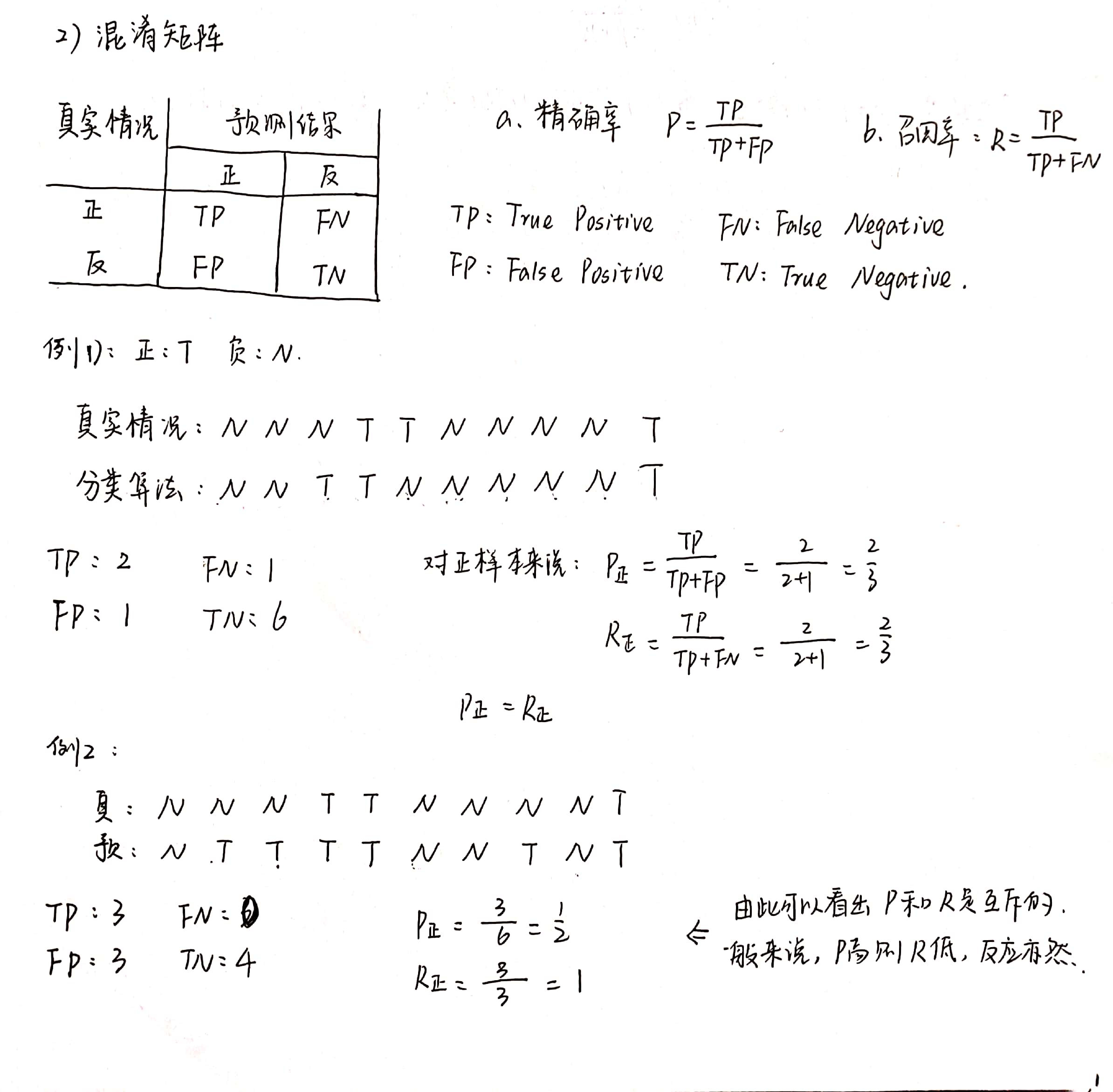3. F1_score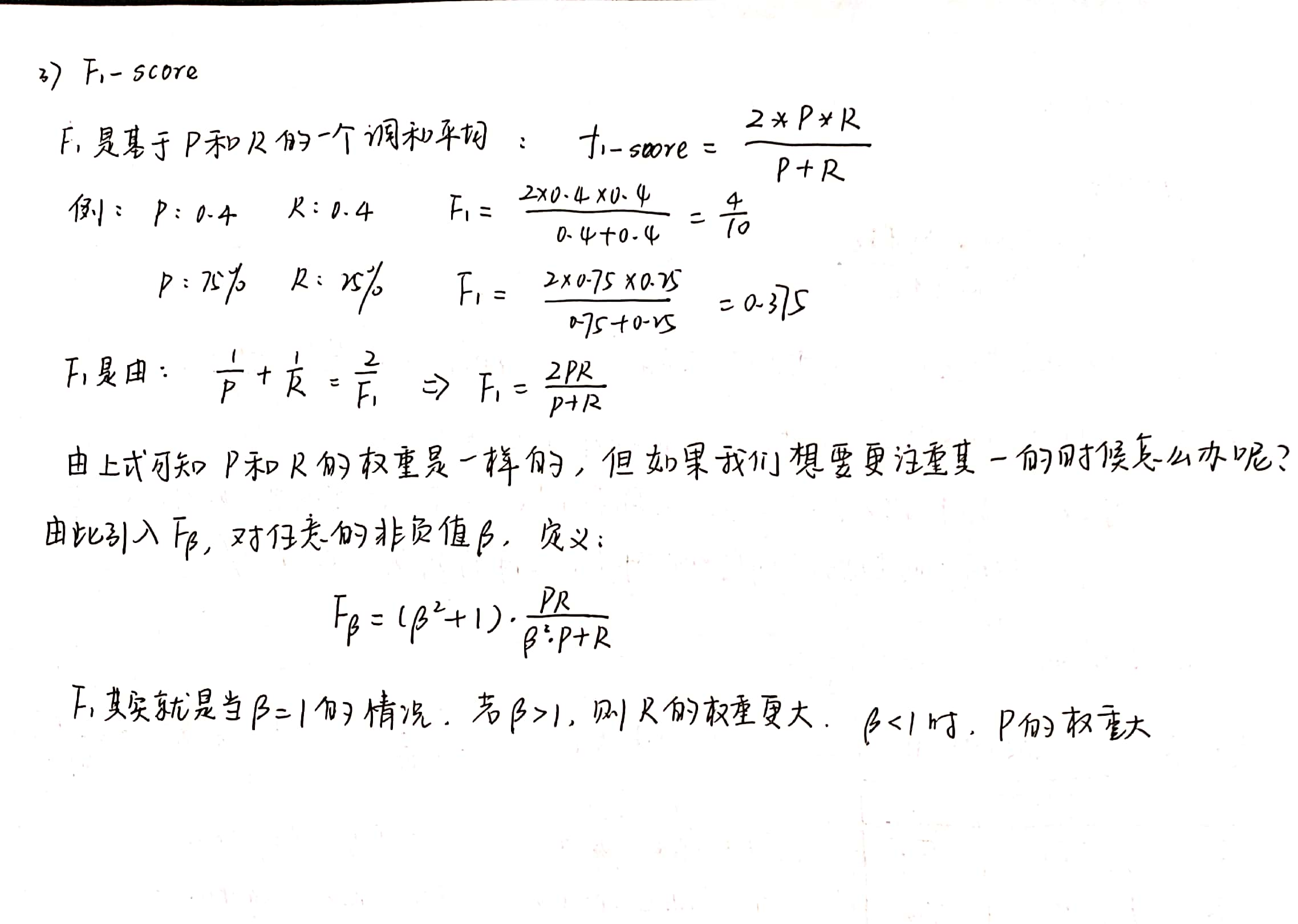举例说明：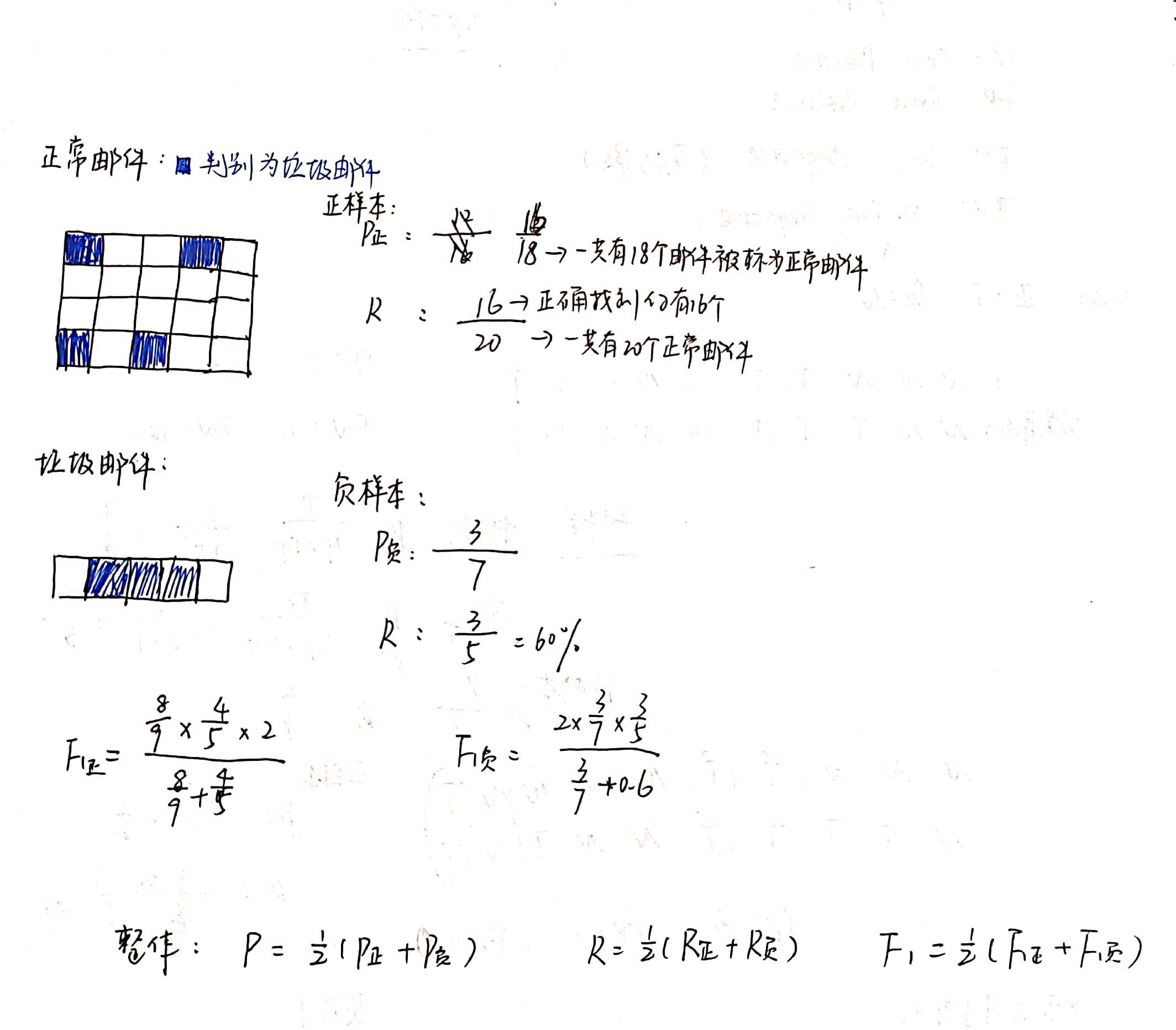4.PR曲线、ROC曲线和AUC
(1）PR曲线
对于同一模型，我们可以通过调整阈值得到不同的P-R值，从而得到一条曲线。（P就是查准率（Precision），R就是查全率（Recall）。以P作为横坐标，R作为纵坐标，就可以画出P-R曲线。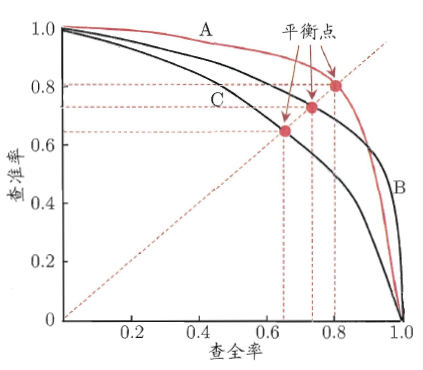当我们比较两个分类器好坏时，P和R都是越大越好也就是图中的曲线越往坐标（1，1）的位置靠近越好。如果一个学习器的P-R曲线被另一个学习器完全”包住”，则后者的性能优于前者。当存在交叉时，可以计算曲线围住面积，不太容易判断，但是可以通过平衡点（查准率=查全率，Break-Even Point，BEP）来判断。例如上图，基于BEP的比较，可以认为A优于B。
（2）ROC曲线和AUC
AUC（Area Under the ROC Curve）指标在模型评估阶段常被用作最重要的评估指标来衡量模型的准确性。AUC作为模型评价指标，用于二分类模型的评价。AUC考虑的是模型预测的排序质量，反映了模型把正例排在反例前面的比例（如果AUC=1，说明模型100%将所有正例排在反例前面）
真正例率，True Positive Rate：TPR = TP/ (TP+FN)
假正例率， False Postive Rate：FPR = FP/(TN+FP)
真正率，正确预测到的正例数与实际正例数的比值（灵敏度）
特异度 ，正确预测到的负例数与实际负例数的比值（NPV = TN / (TN+FN)）
将假正例率作为横轴，真正例率作为纵轴，可以得到ROC曲线，而AUC则是ROC曲线下的面积。如下图所示：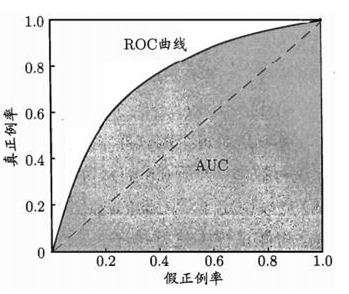对于ROC的图，有几比较重要的点和线：

[0,0]，FPR=TPR=0,  由公式可以看出，TP=FP=0,也就是说模型把所有的样本预测为负例。
[0,1],  FPR=0,TPR=1.  此时FP=FN=0,也就是说模型做出了没有任何错误的完美预测(由此可以看出ROC曲线越是向上凸(贴近上边界),曲线上的点的纵坐标(TPR)越是大,代表模型的性能越好)。
[1,0],  FPR =1,TPR=0.  此时TP=TN=0, 也就是说模型做出的预测全部错误(由此可以看出,ROC曲线越是贴近下边界(下凹),那么模型的性能越是差)。
[1, 1]，FPR =1,TPR=1. 此时TN=FN=0,也就是模型将所有样本均预测为正例。
对角线：在对角线上的点都有TPR = FPR, 也就是说对于任意抽取的样本,模型将其中正例预测为正例的概率和将负例预测为正例的概率是相等的.这也就意味着和随机分类的性能一样.
对角线左上方的曲线： 一般情况下，机器学习模型要优于随机分类器,因此我们经常看到的曲线都是像图中的ROC曲线.它们相比对角线是有明显上凸的,同样的横坐标(FPR)下,ROC线是大于对角线的,即代表模型将正例预测为正例的概率要大于将负例预测为正例的概率。所以如果模型越往左上方凸，那么效果越好。

AUC：对于任意一对正负例样本,模型将正样本预测为正例的可能性大于将负例预测为正例的可能性的概率。其数值大小(越大越好)代表了模型的性能优劣。
例如: AUC = 0.5, ROC曲线为对角线时, 模型将一对正负例样本中的正样本预测为正例的概率为0.5,将负样本预测为正例的概率也为0.5,相当于于随机分类预测)  AUC=0.7，对角线左上方的曲线，其含义可以理解为：模型将一对正负例样本中的正样本预测为正例的概率为0.7。

AUC = 1:全部分类正确
0.5 < AUC < 1，优于随机分类器
0 < AUC < 0.5，差于随机分类器

那么为什么要用AUC作为二分类模型的评价指标呢？为什么不直接通过计算准确率来对模型进行评价呢？
因为机器学习中的很多模型对于分类问题的预测结果大多是概率，即属于某个类别的概率，如果计算准确率的话，就要把概率转化为类别，这就需要设定一个阈值，概率大于某个阈值的属于一类，概率小于某个阈值的属于另一类，而阈值的设定直接影响了准确率的计算。也就是说AUC越高说明阈值分割所能达到的准确率越高。
AUC的计算方式:
1、绘制出ROC曲线,然后计算出曲线下包绕的面积,面积值即AUC.
2、假设总共有（T+N）个样本，其中正样本T个，负样本N个，总共有TN个样本对，计数，正样本预测为正样本的概率值大于负样本预测为正样本的概率值记为1，累加计数，然后除以（TN）就是AUC的值


展开全文机器学习
• 本篇文章主要介绍机器学习中常见的指标，由于这些东西很基本，又很常用，所以本篇文章记录下来，加深一下印象。 几种分类 对于一个模型输出的预测结果，一般的将它们分为四个类别：TP、FP、TN、FN 利用下面一张图...
基本评价指标解释
本篇文章主要介绍机器学习中常见的指标，由于这些东西很基本，又很常用，所以本篇文章记录下来，加深一下印象。
几种分类
对于一个模型输出的预测结果，一般的将它们分为四个类别：TP、FP、TN、FN
利用下面一张图可以清晰明了、简单粗暴的解释上面的四个名词：

预测
预测

P
N

实际
P
TP
FN

实际
N
FP
TN

评价指标
准确率(accuracy)
acc = (TP + TN) / total
精确率(precision)
p = TP / (TP + FP)
召回率(recall)
r = TP / (TP + FN)
F1(F-Measure)
通常情况下，精确率和召回率是此消彼长的，所以需要综合考虑，常见的方法就是F-Measure，它是Precision和Recall的加权调和平均
F = ((a^2 + 1) * P * R) / (a^2 * (P + R))
当a=1是，我们就叫F1值：
F1 = (2 * P * R) / (P + R)
并交比(IOU)
IOU = TP / (TP + FP + FN)
参考文献
一张图理解什么是TP、FN、FP、TN
评价指标对比：准确率(accuracy)、精确率(Precision)、召回率(Recall)、IOU、Kappa系数


展开全文自然语言处理
• 文章目录分类模型的评价指标回归模型的评价指标 分类模型的评价指标 首先区分几个基本概念： TP(True Positives)：预测为正样本，实际上也为正样本样本数； FP(False Positives)：预测为正样本，实际上为负样本...
文章目录分类模型的评价指标回归模型的评价指标
分类模型的评价指标

首先区分几个基本概念：

TP(True Positives)：预测为正样本，实际上也为正样本的样本数；
FP(False Positives)：预测为正样本，实际上为负样本的样本数；
TN(True Negatives)：预测为负样本，实际上也为负样本的样本数；
FN(False Negatives)：预测为负样本，实际上为正样本的样本数。
通过下面的图片可以直观的理解这几个概念。准确率(Accuracy)。所有预测正确的样本占总样本的比例。
$Accuracy=\frac{TP+TN}{TP+FP+TN+FN}$
精确率(Precision)。正确的预测为正的样本占所有预测为正的样本的比例。
$Precision=\frac{TP}{TP+FP}$
TPR(True Positive Rate)。正确预测为正的样本占所有正样本的比例，即在所有真实正样本中被正确分类的样本比例。
$TPR=\frac{TP}{TP+FN}$
FPR(False Positive Rate)。错误预测为正的样本占所有负样本的比例，即在所有负样本中被错误分类的比例。
$FPR=\frac{FP}{FP+TN}$
召回率(Recall)。
$Recall=\frac{TP}{TP+FN}$
知道了TPR和FPR的概念后，就很容易得到ROC(Receiver operating characteristic)曲线，ROC曲线是以TPR为纵坐标，以FPR为横坐标形成的曲线，如下图所示。图中由ROC曲线包围的面积称为AUC(Area under the ROC Curve)。AUC的值介于0和1之间，可以等于0或者1,AUC的值越大表示模型的分类效果越好。

这是一个很好的ROC曲线的解释：Understanding ROC curves

Log loss
Log loss又称作 logistic regression loss 或者 cross-entropy loss，Sklearn中使用自然对处e为底。对于二分类问题，样本真实标签$y\in\{-1,1\}$，概率$p=Pr(y=1)$，则每个样本的对数损失为：
$L_{log}(y,p)=-\log Pr(y|p)=-(y\log p+(1-y)\log (1-p))$
所有样本的对数损失为：
$L_{log}=-\frac{1}{N}\sum_{i=1}^{N}[y_i\log p_i+(1-y_i)\log(1-p_i)]$
公式中的负号是为了让最大似然值和最小损失对应起来。因为$0\leq p \leq1$，$p$越接近1表示预测正确的概率越大，损失应该越小。而$log$函数在0到1之间是小于0的单调递增函数，增加负号则变成0到1之间大于0的单调递减函数，$p$越接近1则$-\log p$ 越小，损失也越小。
对于多分类问题的log loss计算公式如下：
$L_{log}(Y,P)=-\log Pr(Y|P)=-\frac{1}{N}\sum_{i=1}^{N-1}\sum_{k=0}^{K-1}y_{i,k}\log P_{i,k}$
其中$N$表示样本数，$K$表示样本标签数。如果第$i$个样本标签为$k$，则$y_{i,k}=1$，$P_{i,k}$表示对应的概率。
以Sklearn中的例子说明：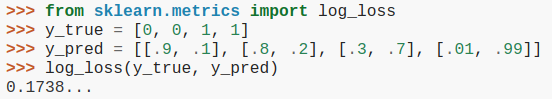log_loss函数计算损失等价于：

import numpy as np
logloss = -(np.log(0.9) + np.log(0.8) + np.log(0.7) + np.log(0.99)) / 4

以上是二分类问题，log_loss函数也可用于计算多分类问题：
y_true = [0, 0, 1, 1, 2, 2]
y_pred = [[.8, .1, .1], [.8, .1, .1], [.3, .6, .1], [.01, .89, .1], [.1, .1, .8], [.1, .2, .7]]
logloss = log_loss(y_true, y_pred)

logloss = -(np.log(.8) + np.log(.8) + np.log(.6) + np.log(.89) + np.log(.8) + np.log(.7)) / 6

可以验证，上面两个代码块得出的损失值相同。
回归模型的评价指标

Mean Absolute Error(MAE)，平均绝对误差。
$MAE(y,\hat{y})=\frac{1}{n}\sum_{i=0}^{n-1}|y_i-\hat{y_i}|$
其中$n$表示样本数，$y_i$表示第$i$个样本的真实标签，$\hat{y_i}$表示第$i$个样本的预测值。
Mean Squared Error(MSE)，均方误差。
$MSE(y,\hat{y})=\frac{1}{n}\sum_{i=0}^{n-1}(y_i-\hat{y})^2$
Root Mean Squared Error(RMSE)，均方根误差。
$RMSE(y,\hat{y})=\sqrt{\frac{1}{n}\sum_{i=0}^{n-1}(y_i-\hat{y})^2}$
Mean Squared Logarithmic Error(MSLE)，均方对数误差。
$MSLE(y,\hat{y})=\frac{1}{n}\sum_{i=0}^{n-1}(\log(1+y)-\log(1+\hat{y}))^2$


展开全文机器学习 分类和回归
• 分类模型的性能评价指标，主要包含准确率，精确率、召回率、f1_score，ROC曲线，AUC等 1、分类评价指标 先列出混淆矩阵 其中： TP：真实值是positive，模型分为positive FN：真实值是positive，模型分为negative FP...
分类模型的性能评价指标，主要包含准确率，精确率、召回率、f1_score，ROC曲线，AUC等
1、分类评价指标
先列出混淆矩阵其中：
TP：真实值是positive，模型分为positive
FN：真实值是positive，模型分为negative
FP：真实值是negative，模型分为positive
TN：真实值是negative，模型认为是negative
1.1、准确度（Accuracy）
准确度：对于给定的测试集，模型正确分类的样本数与总样本数之比。
公式为：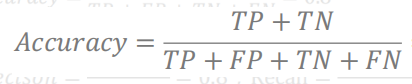1.2、精确度（precision）
精确度：对于给定测试集，分类模型将正类样本预测为正类的数量与将样本预测为正类的综述的比例。
公式：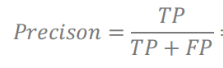1.3、召回率（Recall）
召回率：对于给定测试集，模型将正类样本分为正类的数量与，模型分类正确的数量的比值。
公式：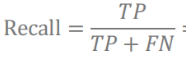1.4、F_score
该指标用来综合的衡量模型的召回率和精确度
公式：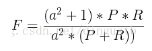其中，a表示权重因子，P（precision）表示精确度，R（recall）表示召回率
当a = 1 时，便是F1_score
F1_score公式：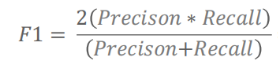1.5、Matthews相关系数（Matthews correlation coefficient，MCC）
MCC：用一个值综合混淆矩阵，度量真实值和预测值之间的相关性。
分母中任意一对括号相加之和如果为0，那么整个MCC的值就为0。
公式：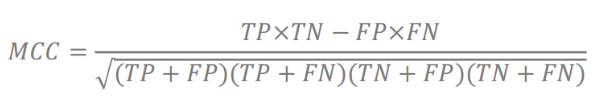MCC值在[-1,1]之间：
1：分类器是完美的
0：分类器是随机分类器
-1：分类器是最差，所有预测结果和实际相反
1.6、AUC（Area under the ROC curve）
ROC曲线一定程度上可以反映分类器的分类效果。AUC作为一个模型分类好坏的评价指标，能够直观地反映了ROC曲线表达的分类能力。AUC实际上就是ROC曲线下的面积。
AUC = 1采用这个预测模型时，不管设定什么阈值都能得出完美预测。绝大多数预测的场合，不存在完美分类器。
0.5 < AUC < 1，优于随机猜测。这个分类器（模型）妥善设定阈值的话，能有预测价值。
AUC = 0.5，跟随机猜测一样（例：抛硬币），模型没有预测价值。
AUC < 0.5，比随机猜测还差；但只要总是反预测而行，就优于随机猜测，因此不存在AUC < 0.5的情况。


展开全文机器学习
• 机器学习-分类模型常用评价指标二分类模型多分类模型详见参考文章 最常见的指标Accuracy到底有哪些不足。 Accuracy是分类问题中最常用的指标，它计算了分类正确的预测数与总预测数的比值。但是，对于不平衡数据集而...机器学习
• 1. 基本概念 ...TP —— True Positive （真正, TP）被模型预测为正正样本；...TN —— True Negative（真负 , TN）被模型预测为负负样本 ；可以称作判断为假正确率  FP ——False Positive
• 在此总结一下基本的模型评价指标和使用方法： 附上几个写比较清晰博客： 常见回归评价 一文读懂分类算法常用评价指标 解sklearn中logloss计算过程 评价指标的选择是很重要 回归问题 MSE（均方误差） 真实...
• 要注意，精确率和召回率是二分类指标，不适用多分类，由此得到P-R曲线以及ROC曲线均是二分类评估指标（因为其横纵轴指标均为二分类混淆矩阵计算得到），而准确率适用于多分类评估。（可以将多分类问题转换为二分类...机器学习 深度学习
• 对于回归而言，更注重模型拟合曲线，相对于真实曲线误差。主要包括：拟合优度/R-Squared，校正决定系数(Adjusted R-square)，均方误差（MSE），均方根误差（RMSE），...MSE可以评价模型的预测精度，MSE值越机器学习
• 在预测任务中，给定样例集D={(x1,y1),(x2,y2),…,(xm,ym)}D=\lbrace(x_1,y_1),(x_2,y_2),\ldots,(x_m,y_m)\rbraceD={(x1​,y1​),(x2​,y2​),…,(xm​,ym​)}，其中yiy_iyi​是示例xix_ixi​真实标记，mmm表示...机器学习
• TP —— True Positive （真正, TP）被模型预测为正正样本；可以称作判断为真正确率 TN —— True Negative（真负 , TN）被模型预测为负负样本 ；可以称作判断为假正确率 FP ——False Positive （假正, ...
• 一、模型评价的意义 在完成模型构建之后，必须对模型的效果进行评估，根据评估结果来继续调整模型的参数、特征或者算法，以达到满意的结果。 评价一个模型最简单也是最常用的指标就是准确率，但是在没有任何前提下...
• 有很多指标可以衡量机器学习模型的效果，不同任务使用的评价指标也不尽相同。本文对二分类任务的评价指标加以总结。全文系作者原创，仅供学习参考使用，转载授权请私信联系，否则将视为侵权行为。码字不易，感谢...机器学习 二分类
• 当一个模型构建完成之后，我们可以通过几个不同性能指标来衡量分类模型的相关性能，常用的分类性能指标有准确率(precision)、召回率(recall)和F1分数(F1-score)。 一、混淆矩阵 混淆矩阵(confusion matrix):是...ROC
• 在使用机器学习解决实际问题时，通常有很多模型可用。...模型性能的评价常用的指标有：accuracy、precision、recall等。这些指标的选择需要根据应用场景具体而定。对于特定应用，使用合适的评价指标来客观地...mAP 目标检测
• 准确率是一个用于评价分类模型的指标。通俗来说，准确率是指我们的模型预测正确的结果所占的比例。 Accuracy=Number of correct predictions /Total number of predictions 对于二元分类，也可以根据正类别和负...
• 图像分割常用的性能测试指标 图像分割性能评估方法，主要从文本检索中借鉴而来。因为我们可以将分割像素点根据是否有无标记，映射到文本相关性与否上来，这样就可以将文本检索中性能评估引入到图像分割...深度学习
• 均方误差 （Mean Squared Error，MSE） 观测值与真值偏差平方和与观测次数比值： 这就是线性回归中最常用的损失函数，线性回归过程中尽量让该损失...MSE可以评价数据变化程度，MSE值越小，说明预测模型描...
• 回归模型的评价指标 一、总体介绍 1. 均方误差 （Mean Squared Error，MSE） 观测值与真值偏差平方和与观测次数比值： MSE=1m∑i=1m(fi−yi)2MSE = \frac{1}{m}\sum_{i=1}^{m}(f_i-y_i)^2MSE=m1​i=1∑m​(fi​...数据分析
• 小书匠深度学习分类方法常用的评估模型好坏方法. 0.预设问题 假设我现在有一个二分类任务,是分析100封邮件是否是垃圾邮件,其中不是垃圾邮件有65封,是垃圾邮件有35封.模型最终给邮件结论只有两个:是垃圾邮件...
• AUC是一种衡量机器学习模型分类性能的重要且非常常用的指标,其只能用于二分类的情况. AUC的本质含义反映的是对于任意一对正负例样本,模型将正样本预测为正例的可能性 大于 将负例预测为正例的可能性的 概率( :-) 没...数据挖掘 机器学习
• 推荐中常用的模型评价指标有准确率，召回率，F1-score和AUC。 1. 什么是AUC AUC指标是一个[0,1]之间实数，代表如果随机挑选一个正样本和一个负样本，分类算法将这个正样本排在负样本前面概率。值越大，表示分类...
• 模型评价：回归模型的常用评价指标 1） 样本误差：衡量模型在一个样本上预测准确性     样本误差 = 样本预测值 – 样本实际值 2） 最常用的评价指标：均误差方（MSE）     指标解释：所有样本样本误差平方...
• 机器学习算法的常用评价指标一、常用评价指标的介绍二、实例三、结论三、结论 一、常用评价指标的介绍 1、TP、TN、FP、FN介绍 TP:被正确诊断为患病病人 TN:被正确诊断为健康健康人 FP:被错误诊断为患病健康人......